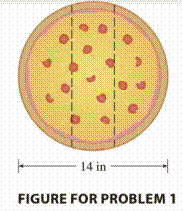Chapter 7.P, Problem 1P

Chapter
Section
Textbook Problem
259 views

# Three mathematics students have ordered a 14-inch pizza. Instead of slicing it in the traditional way, they decide to slice it by parallel cuts, as shown in the figure. Being mathematics majors, they are able to determine where to slice so that each gets the same amount of pizza. Where are the cuts made?To determine

To find:

Where are the cuts are made on the 14-inch pizza to obtain 3 same pizza pieces.

Solution:

The slicing distance is 1.85 inch from the centre.

Explanation

Given:

Pizza size is 14 inch.

Radius of the pizza be R which is 7 inch.

Decided to be cut in parallel way.

Each of the three student to get same amount of pizza piece.

Let the radius of the pizza be R which is 7 inch.

The distance of any general strip from the centre be r,

Length of the strip 2R2r2

Width of the strip dr

Area of the strip 2R2r2dr

Let distance of the slicing from the centre be d,

Integrating from d to R we shall get the area of slice. But it is one third of total area so it is πR23

Thus we have

πR23=2dRR2r2dr=R2cos1dRdR2d2=1.85

Conclusion:

The slicing distance is 1.85 inch from the centre.

#### The Solution to Your Study Problems

Bartleby provides explanations to thousands of textbook problems written by our experts, many with advanced degrees!

Get Started

#### Find more solutions based on key concepts